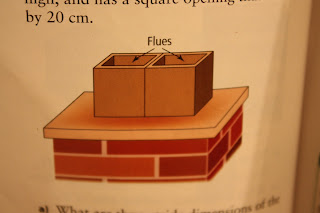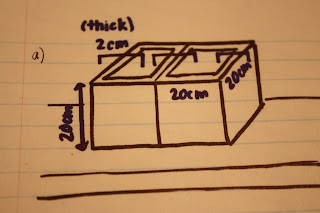Monday, December 17, 2012

Textbook 1.3 Question 23

• Before I looked for the surface area I had to divided the side into a way I could find the surface area easily because the shape is irregular.
• So I divided the shape into two pieces one into a triangle and one into a rectangle.
• First I used the Pythagorean Theorem to find the measurement of the base of the pool.
• After I square rooted my answer that gave me the length of the base.
• Now I can find the surface area of that side.

• Now I started finding the surface area of the inside walls of the pool that need painting which was the bottom of the pool, the side, back and front.
• I found the surface area of the rectangle in the irregular shape then the triangle then added them together, then multiplied it by two.
• Then I found the S.A of the base (bottom of the pool) by multiplying the length and width.
• Next I found the front and the back by using the same formula but different heights because the pool gradually gets greater.
• Then as a result I added all the it together and got an answer of 389 m^2.
*This may not be the answer at the back because I could not find a way to get that answer. (:

Chapter 1,3 Page 34: Question 17Question: The hollow passages through which smoke and fumes escape in a chimney are called flues. Each flue shown is 2cm thick, 20 cm high, and has a square opening that is 20cm by 20 cm.

a) What are the outside dimensions of the two flues?
b) If the height of each flue is 30cm, what is the outside surface area of the two flues? Hint: Do not forget the flat edges at the top.

---------------------------------------------------------------------------------------------------------------------------------

SOLUTION• Since each flue shown is 2cm thick, and the given opening is 20cm by 20cm, we know that it doesn't really give us the width of one flue.
• One flue will be 24cm because you are adding the 2cm from each side and adding the width from the given opening.
• Dimensions are width x height (24cm x 20cm)
• 20cm + 2(2cm) = 24cm
Answer: The outside dimensions of the two flues are 24cm(width) by 20cm(height).

• Before we start, to make it easier we should be able to change the image so it cooperates with the given description for the flue.
• Instead of 20cm, I changed it to 30cm. The length will be 24cm because I used the same method. [ 20cm + 2(2cm) ]
• However, for the width of the two flues together, it is 48cm because I doubled 24cm because there are two flues, not one.
SA= lw + 2( lh+hw ) - 2( lw )
= (24cm)(48cm) + 2 [ (24cm)(30cm) + (30cm)(48cm) ] - 2[(20cm)(20cm)
= 1152cm² + 2( 720cm² + 1440cm² ) - 2(400cm²)
= 1152cm² + 2(2160cm²) - 800cm²
= 1152cm² + 4320cmm² - 800cm²
= 5472cm² - 800cm²
= 4672cm²

Answer: The surface area of the two flues is 4672cm².

---------------------------------------------------------------------------------------------------------------------------------

Textbook: Page 34 #14

14) A chimney has the dimensions shown. What is the outside surface area of the chimney? Give your answer to the nearest hundredth of a square metre.

Lables:
r1 & h1= big cylinder
r2 & h2= small cylinder

SA= 2π(r1xh1) + 2π(r2xh2) + [πr2^2 - πr1^2]
SA= 2π(25)(90) + 2π (15)(40) + [π(25)^2 - π(15)^2]
SA= 14137.17cm^2 + 3769.91cm^2 + [1963.50 - 706.86]
SA=  14137.17cm^2 + 3769.91cm^2 + 1256.64cm^2
SA= 19163.71cm^2

Conversion:
1963.71cm^2 x 1m^2/10000cm^2=1.92m^2

Friday, December 14, 2012

Textbook: Chapter 1.3 - Question #13

13) A mug for hot beverages is to be designed to keep its contents warm as long as possible.
The rate at which the beverage cools depends on the surface area of the container.
The larger the surface area of the mug, the quicker the liquid inside it will cool.

a) What is the Surface Area?
Assume that neither has a lid.

b) Which is the better mug for keeping drinks warm? Justify your answer.

SOLUTION:

To find the Surface Area of both mugs, we need to know what formula to use.
Since these look like CYLINDERS, we must use the formula for a cylinder.

Surface Area of a Cylinder = 2 π r² + 2 π r h

BUT.. there's a catch! In the question, they said assume that there is no lid.
SO.. since there are usually 2 circles in a cylinder, we must remove the area of 1 circle in the formula.
Our formula will change into:  π r² + 2 π r h

Here are my solutions: PART ABlue Mug = 286.52 cm²
Red Mug = 298.64 cm²

PART B

The mug best for keeping drinks warm is the BLUE mug because in the question itself it stated "The larger the surface area of the mug, the quicker the liquid inside it will cool." The surface area of the blue mug is only 286.52 cm ² compared to the red mug that is 298.64 cm ²